## Interpretation/Usage of Random Walk R-Square in PROC UCM

From the SAS Documentation, the information on this metric is sparse:

Random Walk R-square

The random walk R-square statistic (Harvey’s R-square statistic that uses the random walk model for comparison),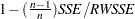, where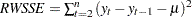, and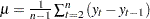From that brief description I've assumed that a positive value would mean that the model fits better than a random walk, and if negative it fits worse. Is that correct? Is the random walk presumed to be non-seasonal (Weekly data, so it's comparing to a random walk where I'd use last week's data rather than last year's data)?

I'm interested in possibly using this as one of our primary metrics as we are producing 100s of forecasts automatically and need some basic diagnostics that we can review. We were using adjusted R2 but with the wide range of possible values (since it can be negative) this is often volatile as we iterate through different model specifications. With some of our series that have significant level shifts, which we capture with a dummy regressor, the adjusted R2 is particularly "poor" (not necessarily true but when you're accustomed to seeing it be b/w 0 and 1) even though the fit looks pretty good, the regressor does it's job, and the forecast is solid.

If I am correct about the interpretation of RW R Sq then this would be a useful metric for model performance as we have already established a precedence with our internal customers on using a naive forecast as a benchmark. I'd also like to determine if it can be a more useful metric for our team's diagnostics to quickly determine if our model tuning process is performing well (We want to see Goodness of Fit improve as we iterate) and flag negative changes over time (Goodness of Fit decreases compared to the last month's forecast).

Example of the distribution of each metric for a sample of our forecasts. RW R Sq has only a few negative values and those may be series we should manually investigate, whereas I cannot come to that conclusion for Adj R Sq. The median for RW R Sq is .35, but .19 for Adj R Sq. I do not want to pick the metric that makes the forecast look better, but I do want to use one that is easier to interpret and give good feedback about the forecast performance.

(Yes, we do also use training data for this as well. But as we refresh the forecast monthly we do not want to reserve training data each time. We plan on periodically doing test forecasts with training data. We have tested our methodology using training data prior to deploying).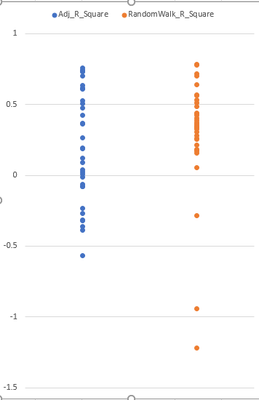1 ACCEPTED SOLUTION

Accepted Solutions

## Re: Interpretation/Usage of Random Walk R-Square in PROC UCM

You are correct.  The random walk R-square (RWRSq say) uses random walk with drift as a baseline model (whereas the usual R-square or its adjusted version use constant as a baseline).  Among the simpler goodness of fit measures, RWRSq is a preferred measure for time series model selection (particularly for time series that are non-stationary).  You are also correct that RWRSq does not take seasonality into account.  Harvey's book (Harvey, A. C. (1989). Forecasting, Structural Time Series Models, and the Kalman Filter. Cambridge: Cambridge University Press.) discusses (see section 5.5.5) yet another R square that does account for seasonality.  It is easy to compute using the one-step-ahead residuals produced by UCM (however, UCM does not output it).

Hope this helps you some.

5 REPLIES 5

## Re: Interpretation/Usage of Random Walk R-Square in PROC UCM

You are correct.  The random walk R-square (RWRSq say) uses random walk with drift as a baseline model (whereas the usual R-square or its adjusted version use constant as a baseline).  Among the simpler goodness of fit measures, RWRSq is a preferred measure for time series model selection (particularly for time series that are non-stationary).  You are also correct that RWRSq does not take seasonality into account.  Harvey's book (Harvey, A. C. (1989). Forecasting, Structural Time Series Models, and the Kalman Filter. Cambridge: Cambridge University Press.) discusses (see section 5.5.5) yet another R square that does account for seasonality.  It is easy to compute using the one-step-ahead residuals produced by UCM (however, UCM does not output it).

Hope this helps you some.

## Re: Interpretation/Usage of Random Walk R-Square in PROC UCM

Thanks. I happened to be near our local university library today and stopped in and copied section 5.5.5 and some more info on goodness of fit from chapter 7. I hope to read it sometime in the next week and then consider what it will take, and the benefits, of potentially calculating this metric on our own.

## Re: Interpretation/Usage of Random Walk R-Square in PROC UCM

I have been working to reverse engineer the PROC UCM RW Rsq calculation (with goal of then calculating the Seasonal version). I started with Harvey and had a difficult time getting the same value as SAS. Then I used the formulas from the SAS/ETS documentation and also struggled.

I am able to reproduce Rsq and Adj Rsq w/in about .01, so I assume my calculation of SSE is correct.

For RW Rsq then my problem was with RWSSE, defined as, and.

Doing that I would consistently get RW Rsq far higher than PROC UCM. However, if I drop the μ term altogether from the RWSSE formula then I do get close to the SAS RW Rsq. The formula for RWSSE is written differently than in Harvey but it appears to be equivalent: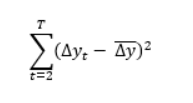I'd like to determine a) if I'm correctly matching PROC UCM and if so, b) which method is preferred so that I extrapolate from there for the seasonal calculation.

## Re: Interpretation/Usage of Random Walk R-Square in PROC UCM

Actual implementation of such formulae is always a little tedious because of various checks for missing values and other issues.  Anyway, here is an illustration.  The data step after the UCM call illustrates the RW R Square computation.  The formula in the doc essentially says that RW R Square = 1 - MSE/RWMSE where MSE is the mean error sum of squares of the residuals and RWMSE is the mean error sum of squares based on the first differences.  The two quantities could be based on different number of summands based on the missing value patterns.  Moreover, the first differences before the first time the non-missing residuals begin are ignored during the RWMSE computation.  Note that this is only a rough illustration.  The actual internal code might have more checks and other niceties.

/*------------------------------------------------*/

proc ucm data=sashelp.air;
id date interval=month;
model air;
irregular;
level;
slope;
estimate;
run;

data fit;
set for(keep=date air residual);
retain sse 0; /* residual sum of squares */
retain rwss rwsum 0; /* for RWSSE computation */
retain nobs1 nobs2 0; /* count of summands */
retain firstNonMiss 0; /* First non-missing residual encountered */
dair = dif(air);
if residual ^= . then do;
sse = sse + residual*residual;
nobs1 = nobs1 + 1;
firstNonMiss = 1; /* remains 1 */
end;
if dair ^= . & firstNonMiss = 1 then do;
rwsum = rwsum + dair;
rwss = rwss + dair*dair;
nobs2 = nobs2 + 1;
end;
if date = '01dec1960'd then do;
/*rwsse based on sum[(x - mean(x))^2] = sum(x^2) - sum(x)*sum(x)/n */
rwsse = rwss - rwsum*rwsum/nobs2;
MRWSse = rwsse/nobs2;
MSE = sse/nobs1;
RWRSq = 1 - MSE/MRWSse;
output;
end;
run;
proc print data=fit;
var nobs1 nobs2 MSE MRWSse rwrsq;
run;

## Re: Interpretation/Usage of Random Walk R-Square in PROC UCM

This was helpful in that I could reproduce in Excel (where I was doing the calculations up to now). I get what you're saying about the missing values, and we usually have several-- I'm just going to ignore any possible difference in nobs1 and nobs2 and just use one N equal to the number of non-missing values of the forecast variable.

I'm going to implement with these calculations and then as a simple seasonal version substitute the prior year value for the same week in the place of the lagged value. This may not be quite the same as Harvey's ("sum of squares of first differences around the seasonal means") but it accomplishes what I'm hoping to do which is to use a less-naive random walk value for RW Rsq metric so that we can conservatively interpret a value above zero as a forecast that is adding value to the process.

Thanks again!

Discussion stats
• 5 replies
• 1982 views
• 0 likes
• 2 in conversation